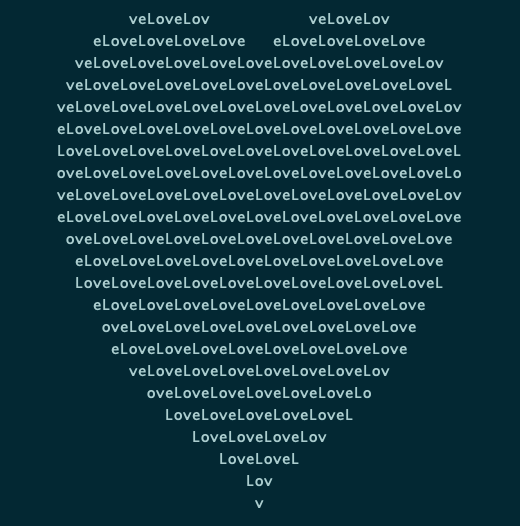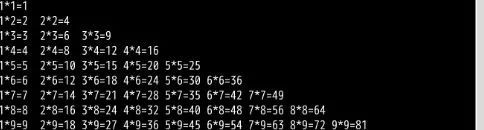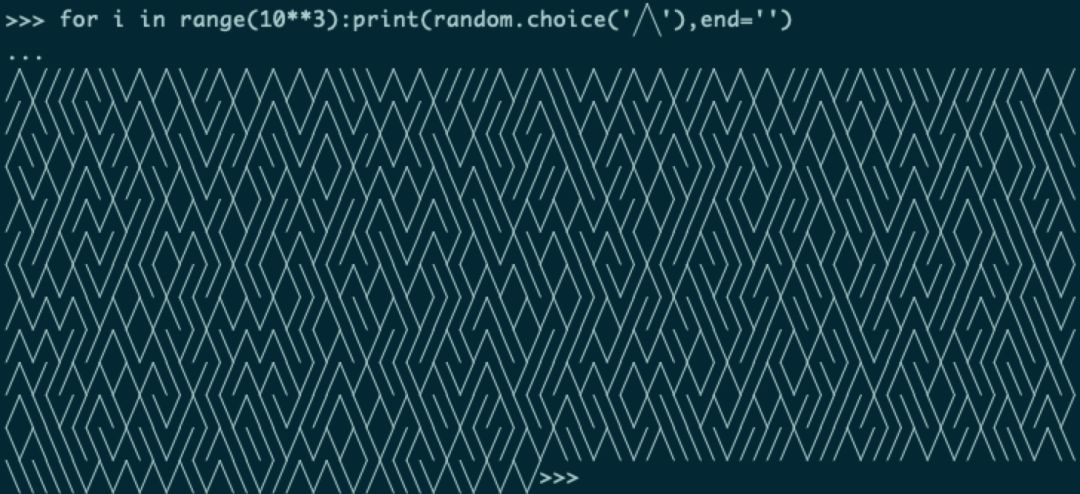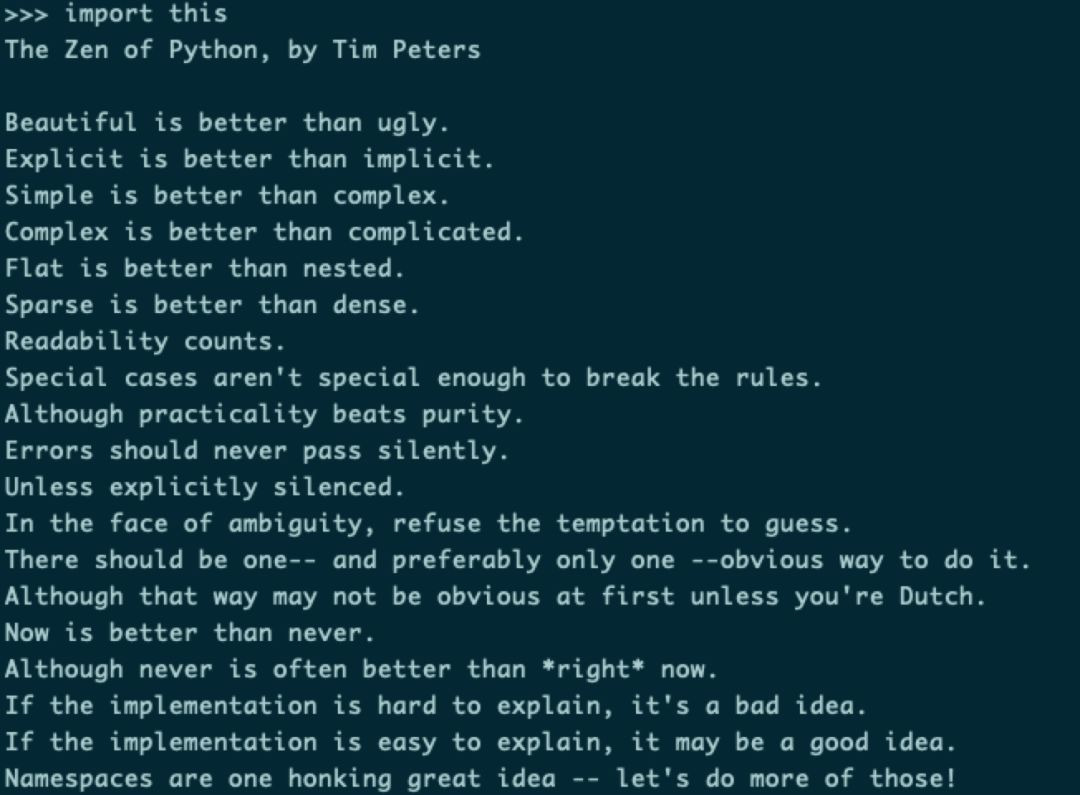2021
08-16

# 你知道一行python语言代码可以干什么吗？

“一行 Python 代码可以干什么？

“可以表白啊！
```
print('\n'.join([''.join([('love'[(x-y) % len('Love')] if ((x*0.05)**2+(y*0.1)**2-1)**3-(x*0.05)**2*(y*0.1)**3 <= 0 else ' ') for x in range(-30, 30)]) for y in range(30, -30, -1)]))

```“啧啧啧……太娘了，有没有刚一点的？”
“一键被全公司人追杀 —— ”
```
git push -f

```
“这……”“还可以一键送自己坐牢！
```
rm -rf /

```
“……有没有积极向上点儿的？”

“一行打印九九乘法表！

```
print('\n'.join([' '.join(['%s*%s=%-2s'%(y,x,x*y)for y in range(1,x+1)]) for x in range(1,10)]))

``````
netsh int tcp set global autotuninglevel=disabled

```

```
for i in range(10**3):print(random.choice('╱╲'),end='')

````python -m http.server`